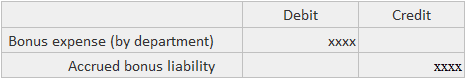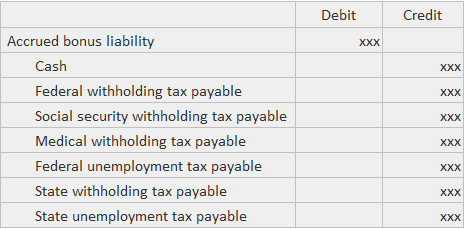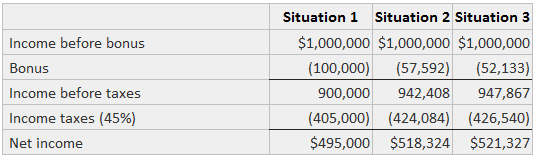A special type of accrued liability arises when a firm agrees to pay a bonus to management contingent upon operating results. There are no substantive conceptual problems as to the classification or disclosures to be provided for liabilities created by these plans.

The only difficulty lies in the calculation of the amount of the bonus under different definitions of the base income to which the bonus rate is applied. Three situations can be identified where the bonus is computed differently.

## Sample Bonus AccrualAccrual bonus paid later:## Bonus Accrual Equations

The general solution technique is to create a set of simultaneous equations that can be solved to determine the size of the bonus. The following symbols are used:

• b = bonus rate
• B = amount of the bonus
• t = income tax rate
• T = amount of income tax
• Y = income before tax and bonus

## Calculation of Bonus Accrual

Situation 1: The bonus is based strictly on pre-bonus income. The equations are:

B = bY
T = t(Y – B)

Situation 2: The bonus is based on pre-bonus income less taxes. The equations are:

B = b(Y – T)
T = t(T – B)

Situation 3: The bonus is based on income after taxes and after the bonus. The equations are:

B = b(Y – T – B)
T = t(Y – B)

Solutions to these three situations are presented below, assuming that:

• b = 10%
• t = 45%
• Y = \$1,000,000

Solution 1:

B = (.10)(1,000,000) = 100,000
T = (.45)(1,000,000 – 100,000) = \$405,000

Solution 2:

B = (.10)(1,000,000 – T)
B = 100,000 – .1T)
T = .(45)(1,000,000 – B)
T= 450,000 – .45B
T = 450,000 – .45(100,000 – .1T)
T = 450,000 – 45,000 + .045T
.955T = 405,000
T = \$424,084
B = 100,000 – (.10)(424,084)
B = 100,000 – 42,408
B = \$57,592

Solution 3:
B = (.10)(1,000,000 – B – T)
10B = 1,000,000 – B – T
T = 1,000,000 – 11B
T = .45(1,000,000 – B)
1,000,000 – 11B = 450,000 – .45B
10.55B = 550,000
B = \$52,133
T = .45(1,000,000 – 52,133)
T = \$426,540

The following table summarizes the results.### What is meant by bonus accrual?

A special type of accrued liability arises when a firm agrees to pay a bonus to management contingent upon operating results. There are no substantive conceptual problems as to the classification or disclosures to be provided for liabilities created by these plans.

### What is the calculation of bonus accrual when it is based strictly on pre-bonus income?

The equations of bonus accrual when it is based strictly on pre-bonus income are: b = by t = t(y – b)

### What is the calculation of bonus accrual when it is based on pre-bonus income less taxes?

The equations of bonus accrual when it is based on pre-bonus income less taxes are: b = b(y – t) t = t(t – b)

### What is the calculation of bonus accrual when it is based on income after taxes and after the bonus?

The equations of bonus accrual when it is based on income after taxes and after the bonus are: b = b(y – t – b) t = t(y – b)

### What symbols are used in bonus accrual equations?

The following symbols are used in bonus accrual equations: b = bonus rate b = amount of the bonus t = income tax rate t = amount of income tax y = income before tax and bonus

True is a Certified Educator in Personal Finance (CEPF®), a member of the Society for Advancing Business Editing and Writing, contributes to his financial education site, Finance Strategists, and has spoken to various financial communities such as the CFA Institute, as well as university students like his Alma mater, Biola University, where he received a bachelor of science in business and data analytics.

To learn more about True, visit his personal website, view his author profile on Amazon, his interview on CBS, or check out his speaker profile on the CFA Institute website.